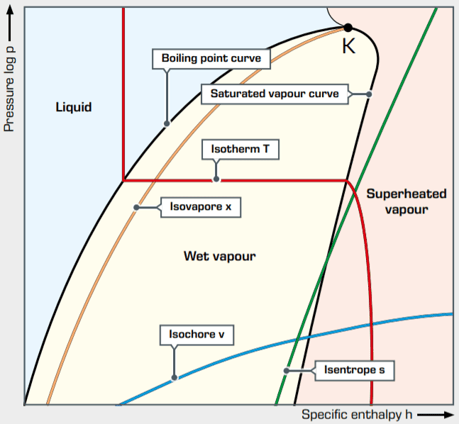# Refrigeration Cycle Diagram Explained

The thermodynamic processes in the refrigeration cycle are complex. Calculation using formulae and tables requires a considerable amount of effort due to the three different states of the refrigerant from liquid, boiling and gaseous. Therefore, for reasons of simplify cation, the log p-h diagram was introduced.

## Refrigeration cycle concept

In general, a log p-h diagram shows the aggregate state of a substance, depending on pressure and heat. For refrigeration, the diagram is reduced to the relevant regions of liquid and gaseous as well as their mixed form.log p-h diagram shows the thermodynamic state variables in the respective phase

The vertical axis shows the logarithmic pressure and the horizontal axis shows the specific c enthalpy with linear scaling. Accordingly, the isobars are horizontal and the isoenthalps are vertical. The logarithmic scaling makes it possible to represent processes with large pressure differences.

The saturated vapor curve and the boiling point curve meet at the critical point K.

• pressure p
• specific enthalpy h
• temperature T
• specific volume v
• specific entropy s
• gas content x

## Log p-h diagram

The distinctive feature of the refrigeration cycle is that it runs counter-clockwise, i.e. opposite to the joule or steam cycle. A change of state occurs when the refrigerant flows through one of the four main components of the refrigeration plant. The actual refrigeration cycle consists of the following changes of state:

• Green = compressor
• Red = condenser
• Yellow = expansion valve
• Blue = evaporator
• 1 – 2 polytropic compression to the condensing pressure (for comparison 1 – 2’ isentropic compression)
• 2 – 2’’ isobaric cooling, deheating of the superheated vapour
• 2’’ – 3’ isobaric condensation
• 3’ – 3 isobaric cooling, supercooling of the liquid
• 3 – 4 isenthalpic expansion to the evaporation pressure
• 4 – 1’ isobaric evaporation
• 1’ – 1 isobaric heating, superheating of the vapour

## Specific amounts of energy

The specific amounts of energy that can be absorbed and released to reach the state points are marked as lines in the log p-h diagram. The specific enthalpy h can be read for each separate state point directly from the log p-h diagram.

If the mass flow rate of the refrigerant is known, the associated thermal output can be calculated by means of the specific enthalpy at the respective state point.

• the line h1 – h4 = q0 corresponds to the cooling and results in the refrigeration capacity by multiplication with the the mass flow rate.
• the line h2 – h1 = pv corresponds to the technical work of the compressor, which is actually transferred to the refrigerant.
• the line h2 – h3 = qc corresponds to the emitted heat and results in the condenser capacity by multiplication with the the mass flow rate. It is the waste heat from a refrigeration plant.

## Limiting isobars

• p1 evaporation pressure
• p2 condensing pressure

## Compression process

• identifying the point of intersection of the isobars p1 with the temperature at the compressor inlet T1 gives the state point 1.
• identifying the point of intersection of the isobars p2 with the temperature at the condenser inlet T2 gives the state point 2.
• the connection between the two state points 1 and 2 describes the compression process

## Isenthalpic expansion

identifying the point of intersection of the isobars p2 with the temperature T3 at the condenser outlet gives the state point 3.

The expansion is an isenthalpic process. Therefore, the previously marked intersection point can be connected to the isobars p1 by a vertical line. This results in the last state point 4 with the evaporation temperature T4

## Reveal the specific enthalpy values

When calculating operating states of a refrigeration plant, it is necessary to determine the specific enthalpies of the individual changes of state. The procedure is as follows:

The specific enthalpy can be read off using a vertical connection of the state points and the x-axis.

• h1 spec. enthalpy after evaporator
• h2 spec. enthalpy after compressor
• h3 spec. enthalpy after condenser
• h4 spec. enthalpy after expansion valve

The specific refrigeration capacity q0 and the specific condensation capacity qc can be read directly from the log p-h diagram.

specific refrigeration capacity q0 = h1 – h4

specific condensation capacity qc = h2 – h3